# NAMING POLYHEDRA

ACTIVITY SHEET

The exercises contained in this activity require the KaleidoTile program developed by Jeff Weeks. If you do not yet have a copy of this software, you may wish to download KaleidoTile .

For more information regarding this activity and its objectives, see the accompanying Teacher's Guide.

1. Get models of the five Platonic solids to study. Each Greek prefix in the table below represents a number. Name each Platonic solid shown in the lower table by:

• counting the number of faces,
• find the Greek prefix that matches the number of faces,
• attach this prefix to "hedron".
• For example, a polyhedron with 5 faces could be called pentahedron.

```Table: Greek Prefixes
Prefix       Number     Prefix          Number
mono         1          hendeca         11
di           2          dodeca          12
tri          3          trideca         13
deca        10          icosa           20
```
```Table: Names of the Platonic Solids
Platonic Solid          Number of Sides         Name
```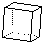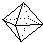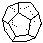2. Look at the sketches of polyhedra that you made from the Intro. to KaleidoTile lesson. Check the names you gave to the Platonic solids above with the names given to your sketches from KaleidoTile. Are any of the names different?

If so, explain why.

3. Load the KaleidoTile program on your computer. You will study one polyhedra family, called the (2,3,4)-family. To do so, choose the settings shown here: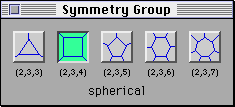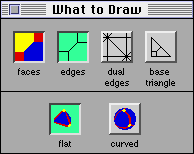• In the Basepoint window, drag the base point to each vertex dot and write the name of the polyhedron formed in the (2,3,4) -Family Diagram.

(2,3,4)-Family Diagram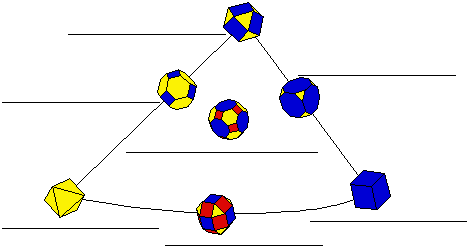• Starting with the base point at the lower right vertex dot, slowly drag the base point along the right edge of the base triangle triangle until you reach the dot in the middle of the edge. Watch as the cube transforms into a new polyhedron. Describe how the polyhedra are changing.

• Continue to drag the base point along the right edge of the base triangle until you reach the upper vertex dot. Again describe how the polyhedra are changing.

• Place your base point at the lower left vertex dot. Slowly drag the base point along the left edge of the base. Describe what happened to the Platonic solid as you moved the base point away.

• Give your own definition of the word truncated.

• Notice that the name of the polyhedron at the top vertex dot is a combination of the names of the two Platonic solids in lower vertices. Why do you think that is?

• Starting at the top vertex, slowly drag the base point straight down toward the dot in the center of the triangle. You are truncating the polyhedron. Pause when you reach the center dot. Then continue until you reach the dot in the middle of the lower edge. Note that these last two polyhedra names have rhombi in them. What do you think rhombi means?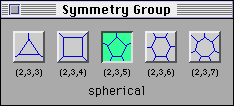4. It's time to check what you learned by studying a different family of polyhedra, the (2,3,5)-family. Change the Symmetry Group by pressing the (2,3,5) button.
• Fill out the (2,3,5)-Family Diagram below by dragging the base point to each vertex dot.

(2,3,5)-Family Diagram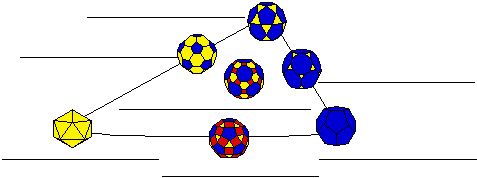• Once again, starting with the base point at the lower right vertex dot, slowly drag the base point along the right edge of the base triangle triangle. Similarly, start at the lower left vertex dot and drag the base point along the left edge. What word describes what is happening in both cases?

• Starting at the top vertex, slowly drag the base point straight down toward the dot in the center of the triangle. Pause when you reach the center dot. Then continue until you reach the dot in the middle of the lower edge. What shapes do these last two polyhedra have that give them rhombi in their name?

• Explain what rhombitruncated icosadodecahedron means.

5. Let's see if you know what is really going on.

• Two names of the (2,3,3)-family are given in the diagram below. Use what you learned from studying the (2,3,4)- and (2,3,5)-families to fill in the other five names. Do NOT use KaleidoTile for this! It will give you incorrect names.

(2,3,3)-Family Diagram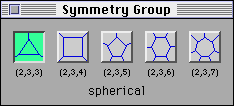• KaleidoTile gives some different names than you should have written. Change the Symmetry Group by pressing the (2,3,3) button. Fill out the (2,3,3)-Family Diagram below, this time using KaleidoTile.
(2,3,3)-Family Diagram• Explain why KaleidoTile named these polyhedra differently than it did for the first two families.

| MAIN | NEXT ACTIVITY | POLYHEDRA CHARTS |